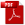Download as PDFOn the number of divisors of a number
Journal of the Indian Mathematical Society, VII, 1915, 131 – 133

1. If $\delta$ be a divisor of $N$, then there is a conjugate divisor $\delta'$ such that $\delta \delta' = N$. Thus we see that

\begin{equation} \mbox{the number of divisors from 1 to } \sqrt{N} \mbox{ is equal to the number of divisors from } \sqrt{N} \mbox{ to } N. \end{equation}

From this it evidently follows that

\begin{equation} d(N) \lt 2 \sqrt{N}, \end{equation}
where $d(N)$ denotes the number of divisors of $N$ (including unity and the number itself). This is only a trivial result, as all the numbers from 1 to $\sqrt{N}$ cannot be divisors of $N$. So let us try to find the best possible superior limit for $d(N)$ by using purely elementary reasoning.

2. First let us consider the case in which all the prime divisors of $N$ are known. Let $$N = p_1^{a_1} \cdot p_2^{a_2} \cdot p_3^{a_3} \cdots p_n^{a_n},$$ where $p_1, p_2, p_3 \ldots p_n$ are a given set of $n$ primes . Then it is easy to see that

\begin{equation} d(N) = (1 + a_1) (1+ a_2) (1+a_3) \cdots (1+a_n). \end{equation}
But
\begin{eqnarray} \frac{1}{n} &&\{(1+a_1) \log p_1 + (1+a_2) \log p_2 + \cdots + (1+a_n) \log p_n \}\notag\\ && > \{(1 + a_1) (1+a_2) \cdots (1+a_n) \log p_1 \log p_2 \cdots \log p_n\}^{\frac{1}{n}}, \end{eqnarray}
since the arithmetic mean of unequal positive numbers is always greater than their geometric mean. Hence $$\frac{1}{n} \{\log p_1 + \log p_2 + \cdots + \log p_n + \log N \} \gt \{\log p_1 \log p_2 \cdots \log p_n \cdot d(N)\}^{\frac{1}{n}}.$$ In other words
\begin{equation} d(N) \lt \frac{\left\{ \frac{1}{n} \log (p_1 p_2 p_3 \cdots p_n N)\right\}^n} {\log p_1 \log p_2 \log p_3 \cdots \log p_n}, \end{equation}
for all values of $N$ whose prime divisors are $p_1, p_2, p_3, \ldots , p_n$.

3. Next let us consider the case in which only the number of prime divisors of $N$ is known. Let $$N = p_1^{a_1} p_2^{a_2} p_3^{a_3} \cdots p_n^{a_n},$$ where $n$ is a given number; and let $$N' = 2^{a_1} \cdot 3^{a_2} \cdot 5^{a_3} \cdots p^{a_n},$$ where $p$ is the natural $n$th prime. Then it is evident that

\begin{equation} N' \leq N; \end{equation}
and
\begin{equation} d(N') = d(N). \end{equation}
But
\begin{equation} d(N') \lt \frac{\left\{\frac{1}{n} \log (2\cdot 3\cdot 5\cdots p\cdot N')\right\}^n} {\log 2 \log 3 \log 5 \cdots \log p} , \end{equation}
by virtue of (5). It follows from (6) to (8) that, if $p$ be the natural $n$th prime, then
\begin{equation} d(N) \lt \frac{\left\{\frac{1}{n} \log(2\cdot 3\cdot 5 \cdots p\cdot N)\right\}^n}{\log 2 \log 3 \log 5 \cdots \log p}, \end{equation}
for all values of $N$ having $n$ prime divisors.

4. Finally, let us consider the case in which nothing is known about $N$. Any integer $N$ can be written in the form $$2^{a_2} \cdot 3^{a_3} \cdot 5^{a_5} \cdots ,$$ where $a_\lambda \geq 0$. Now let

\begin{equation} x^h = 2, \end{equation}
where $h$ is any positive number. Then we have
\begin{equation} \frac{d(N)}{N^h} = \frac{1+a_2}{2^{ha_2}} \cdot \frac{1+a_3}{3^{ha_3}} \cdot \frac{1+a_5}{5^{ha_5}} \end{equation}
But from (10) we see that, if $q$ be any prime greater than $x$, then
\begin{equation} \frac{1+a_q}{q^{ha_q}} \leq \frac{1+a_q}{x^{ha_q}} = \frac{1+a_q}{2^{a_q}} \leq 1. \end{equation}
It follows from (11) and (12) that, if $p$ be the largest prime not exceeding $x$, then
\begin{align} \frac{d(N)}{N^h} &\leq \frac{1+a_2}{2^{ha_2}} \cdot \frac{1+a_3}{3^{ha_3}} \cdot \frac{1+a_5}{5^{ha_5}} \cdots \frac{1+a_p}{p^{ha_p}}\notag\\ &\leq \frac{1+a_2}{2^{ha_2}} \cdot \frac{1+a_3}{2^{ha_3}} \cdot \frac{1+a_5}{2^{ha_5}} \cdots \frac{1+a_p}{2^{ha_p}}. \end{align}

But it is easy to shew that the maximum value of $(1+ a) 2^{-ha}$ for the variable $a$ is $\frac{2^h}{he \log 2}$. Hence

\begin{equation} \frac{d(N)}{N^h} \leq \left(\frac{2^h}{he \log 2}\right)^{\omega(x)}, \end{equation}
where $\omega(x)$ denotes the number of primes not exceeding $x$. But from (10) we have $$h = \frac{\log 2}{\log x}.$$ Substituting this in (14), we obtain
\begin{equation} d(N) \leq N \frac{\log 2}{\log x} \left\{ \frac{2^{\frac{\log 2}{\log x}} \log x}{e(\log 2)^2} \right\}^{\omega(x)} . \end{equation}

But it is easy to verify that, if $x \geq 6.05,$ then $$2^h \lt e (\log 2)^2.$$ From this and (15) it follows that, if $x \geq 6.05,$ then

\begin{equation} d(N) \lt 2^{(\log N)/(\log x)} (\log x)^{\omega(x)} \end{equation}
for all values of $N$, $\omega(x)$ being the number of primes not exceeding $x$.

5. The symbol $O$'' is used in the following sense: $$\phi (x) = O \{(\Psi (x)\}$$ means that there is a positive constant $K$ such that $$\left| \frac{\phi (x)}{\Psi (x)} \right| \leq K$$ for all sufficiently large values of $x$ (see Hardy, Orders of Infinity, pp. 5 et seq.). For example: $$5x =O(x) ; \frac{1}{2} x = O(x); x \sin x = O(x) ; \sqrt{x} = O(x); \log x = O(x);$$ but $$x^2 \neq O(x) ; x \log x \neq O (x).$$

Hence it is obvious that

\begin{equation} \omega(x) = O(x). \end{equation}

Now, let us suppose that $$x = \frac{\log N}{(\log \log N)^2}$$ in (16). Then we have $$\log x = \log \log N + O (\log \log \log N);$$ and so

\begin{equation} \frac{\log N}{\log x} = \frac{\log N}{\log \log N} + O \left\{\frac{\log N \log \log \log N}{(\log \log N)^2}\right\}. \end{equation}
Again
\begin{equation} \omega(x) \log \log x=O (x \log \log x) = O \left\{\frac{\log N \log \log \log N}{(\log \log N)^2} \right\} . \end{equation}

It follows from (16), (18) and (19), that

\begin{equation} \log d (N) \lt \frac{\log 2 \log N}{\log \log N} + O \left\{\frac{\log N \log \log \log N}{(\log \log N)^2} \right\} \end{equation}
for all sufficiently large values of $N$.